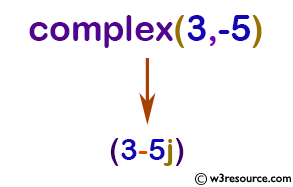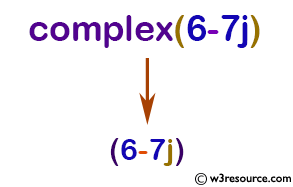# Python: complex() function

## complex() function

The complex() function is used to create a complex number or convert a string or number to a complex number.

The complex type is described in Numeric Types — int, float, complex.

Syntax:

```complex([real[, imag]])
```Version:

(Python 3)

If the first parameter is a string, it will be interpreted as a complex number and the function must be called without a second parameter.

The second parameter can never be a string. Each argument may be any numeric type (including complex).

If imag is omitted, it defaults to zero and the function serves as a numeric conversion function like int() and float(). If both arguments are omitted, returns 0j.

Return value:

Return a complex number with the value real + imag*1j or convert a string or number to a complex number.

Note: When converting from a string, the string must not contain whitespace around the central + or - operator. For example, complex('1+2j') is fine, but complex('1 + 2j') raises ValueError.

Example: Create a complex number in Python

``````x = complex(3, -5)
print(x)
x = complex(1)
print(x)
x = complex()
print(x)
x = complex('6-7j')
print(x)
``````

Output:

```(3-5j)
(1+0j)
0j
(6-7j)
```

Pictorial Presentation:Pictorial Presentation:Example: Create complex Number Without Using complex()

``````x = 3+5j
print('x =',x)
print('Type of x is',type(x))

y = -5j
print('y =',y)
print('Type of y is',type(x))

z = 0j
print('z =',z)
print('Type of z is',type(z))
``````

Output:

```x = (3+5j)
Type of x is
y = (-0-5j)
Type of y is
z = 0j
Type of z is
```

Python Code Editor:

Previous: compile()
Next: delattr()

Test your Python skills with w3resource's quiz

﻿

## Python: Tips of the Day

How do I check if a list is empty?

For example, if passed the following:

```a = []
if not a:
print("List is empty")
```

Ref: https://bit.ly/2A4JXx9